This thread has been locked.

If you have a related question, please click the "Ask a related question" button in the top right corner. The newly created question will be automatically linked to this question.

# OPA627: Noise Bandwidth in multiple stages of OP amps

Part Number: OPA627
Other Parts Discussed in Thread: TINA-TI, THS4131, OPA1632

Hi there,  I am watching TIPL video section 8.4. on page 15 of the slide, when add LPF capacitors, I thinks the Noise BandWidth shoud use Kn=1.22 (rather than 1.57) for U1.

Yes, the noise gain of U2 can be ignored by the simplifying rules, but the Low Pass Filter of U2 (C2 and R4) still workhere. Since f1=f2,  I think it should be a -6dB (2nd order) filter for noise on U1's positive input.Did I misunderstand something?

Further more, a more general question will come out, if U1 and U2 use very different cut-off frequencies, different orders, and even different topology, how should  we determin the order of the filter ?

Thanks!

• Hi Rust,

for the naked single pole low pass filter the factor Pi / 2 = 1.57 should be correct, assuming an ideal OPAmp with infinite bandwidth.

Kai

• Hi Rust,

I am with Kai's point. Since U1 is dominated noise source, 1.57 correction factor may be used because of the 1st order filter.

U2 will introduce another pole in the overall transfer function, but the contributed noise calculation to overall transfer function is not significant as implied in the video. The purpose of the calculation is to estimate the worst case noise errors in the system.

If you have additional questions, please let us know.

Best,

Raymond

• Actually, it seems the truth is in the middle - it is true that there is a double pole at fp=15.9kHz that will cause -6dB gain attenuation instead of -3dB BUT the noise bandwidth is neither BWnoise =1.22*fp (2nd-order) nor BWnoise =1.57*fp (1st-order) because at higher frequency C1 and C2 short across the feedback resistors effectively placing the overall gain at 1 before the unity bandwidth of OPA627 attenuates it further - see AC gain bump inside the red circle below.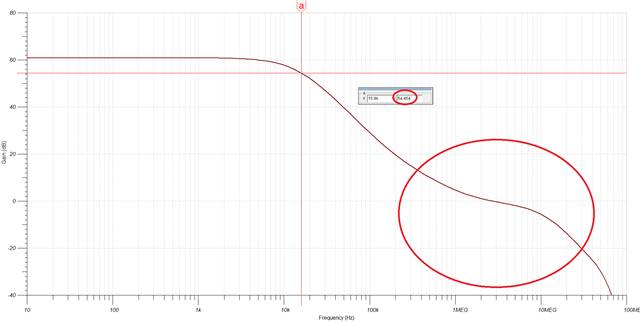For this reason, the rough calculation of the OPA627 noise in TIPL video assumed a worst-case of 1st-order system (green slope below) wheareas the assumption of 2nd-order approximation (red slope below) would under-estimate the integration of noise marked below by the blue vertical lines.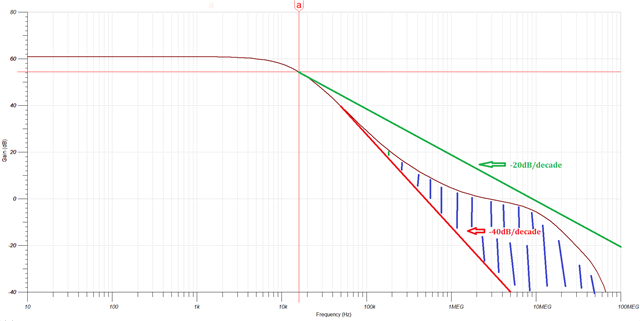The simulation results of the noise spectral density and the total integrated noise of 665uVrms are shown below.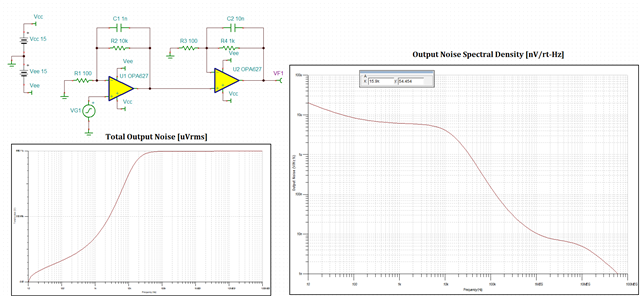For the different cut-off frequencies of multi-stage configuration, you should assume 1st-order system (worst-case) as was done in TIPL video. This will give you rough calculation of the total noise (a sanity check) while you should use simulation tool for more precise total integrated noise estimate.

Attached please find the Tina-TI file you may use for your own noise simulation.

2248.OPA627 Noise.TSC

• Hey Rust, you are on the right track here.

The point of the equivalent noise power BW is to quick calculate the integrated noise without doing the integral. Assuming you care about that to the 2nd stage output, you have the noise output of the 1st stage dominating the equivalent input of the 2nd and getting two poles of rolloff to the output - and yes, 2 repeated real poles is a 1.22X mutiplier to get the equivalent NPBW.

I set this up in sim, and while close I repeatedly get lower integrated noise than I might calculate. At first I thought the added noise in the 2nd stage was making a difference, but that is easy to test and it is not.

Anyway, the OPA627 2019 model up shows about 4.9nV input referred noise. That 4.9nV*101 1st stage gain is 495nV at that output. Then apply the 1.22*15.9kHz or 19.5kHz NPBW for the two stage - take root that times 495nV and then times 11 for the final output integrated noise to get 758uVrms predicted.

Here I ran that and a dependent source emulating but bypassing the 2nd stage noise - that gives the same result showing the noise into the 2nd stage dominates.

I cannot figure out why this is simulating much lower as about 619uVrms. But you are essentially correct, the 2 stages here are 1.22*F-3dB for NPBW.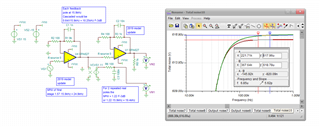• Here is this file,

2stage OPA627 noise.TSC

• Michael,

You get lower total integrated noise, 619uVrms instead of 665uVrms, because you used a different gain in your first stage - R1 of 9k instead of 10k shown in customer schematic (see below).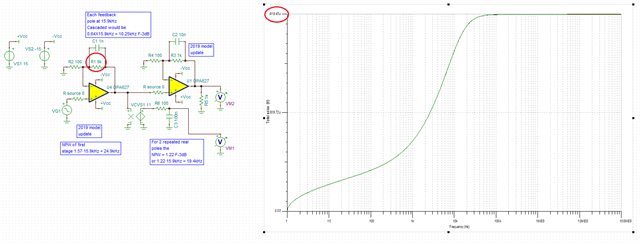• Correct, I changed the Rf in the 1st stage and got closer to the predicted 758uV and yes, Simulating lower is confusing as I would have expected higher since the gain min is 1 here until the GBP is encountered way out.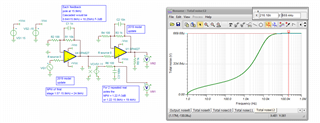• Michael,

Your total noise of 758uVrms using 2nd-order correction factor of 1.22 assumes a broadband noise of 4.9nV/rt-Hz instead of 4.5nV/rt-Hz used by customer.  Using 4.5nV/rt-Hz number results in the total integrated noise of 696uVrms - thus almost exactly what one simulates from 1mHz up - see below.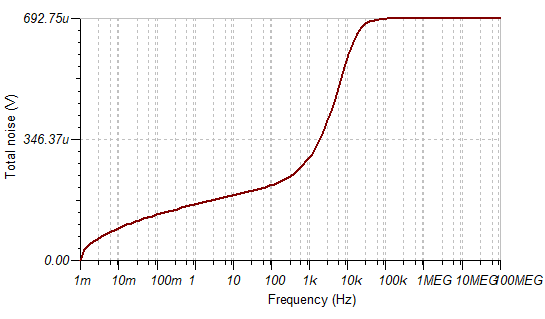• For the different cut-off frequencies of multi-stage configuration, you should assume 1st-order system (worst-case) as was done in TIPL video. This will give you rough calculation of the total noise (a sanity check) while you should use simulation tool for more precise total integrated noise estimate.

Hi Marek, this is the answer I want! and your info in simulation indeed matched with my hand-calculation by writing the transfer function in (jw) domain. It is BETWEEN 1st order and 2nd order.

And also thanks to everyone in this discussion. All answers help a lot!

• And Rust, here is what I was looking at for the 1.22 number, this is a 1982 analysis I was generating for repeated real pole NPBW - the far right column is the ratio of NPBW/F-3dB for each stage. This steps the number of repeated poles up (n) and then gives the equation to continue that ad nauseum at the bottom.

6761.Repeated real pole NPBW.pdf

• I was looking closer at that analysis I sent, oops - the 1.22X factor is times the repeated pole F-3dB, not the individual pole, so try again here,

1st, the output spot noise of the 1st stage gives us what needs to be integrated then gained up in the 2nd and bandlimited more,

THis is actually 5.14nV equivalent input referred or that 519nV at the output. Take this times the sqrt(1.22*10.23kHz) or 112 (that 10.23kHz is the repeated pole F-3dB).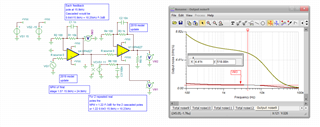519nV*112 = 58uVrms then *11 for the 2nd stage or 639uVrms - this is what the simulation for total output noise should show, So this 669uVrms is only slightly over the anticipated 639uVrms - that make a lot more sense now.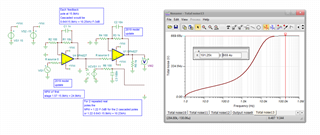• Hi Michael. Thanks for further explain!

By the way, I post a question about FDA noise calculation and measurementas at link below. If you have interest, would you help take a look and share your insight? Thanks again.

• You bet Rust, so actually - going way back to your 1st question, that slide is pretty far off, but - the actual answer might be beyond of the scope of this introductory exposition. I will take a look at that FDA thing.

• Clicking your link on the OPA1632 (which is actually the same die as a the THS4131) shows there is nothing there?

• please try this link, thanks. there was already some discussion with Sima there. You may need some time to go through it if you like. Thanks.

e2e.ti.com/.../opa1632-noise-calculation-for-differential-amplifier